# Tag Archives: formats# Formatting Time Durations in Tableau

This post was updated on 24 July 2015 to include additional details on applying the custom formatting calculation to aggregates.

Here’s a quick lunchtime post on working with durations in Tableau. By duration, I mean having a result that is showing the number of seconds, minutes, hours, and/or days in the form of dd:hh:mm:ss. This isn’t quite a built-in option, there are a several ways to go about this:

• Use any duration formatting that is supported in your data source, for example by pre-computing values or using a RAWSQL function.
• Do a bunch of calculations and string manipulations to get the date to set up. I prefer to avoid these mainly because they can be over 1000x slower than numeric manipulations. If you want to see how to do this, there’s a good example on this Idea for Additional Date Time Number Formats. (If that idea is implemented and marked as Released, then you can ignore this post!)
• If the duration is less than 24 hours (86400 seconds), then you can use Tableau’s built-in date formatting. I’ll show how to do this here.
• Do some calculations and then use Tableau’s built-in number formatting. This is the brand-new solution and involves a bit of indirection.# Durations Guaranteed To Be Less Than 24 Hours: Tableau’s Date Formatting

If you know your duration is always going to be less than 24 hours (86400 seconds), , then you can take advantage of Tableau’s built-in date formatting. The first step is to get your duration in seconds, if it’s not already. For example, for looking at the duration between two dates you can use DATEDIFF(‘second’,[Start Time], [End Time]). Once you have that number of seconds, then you can use the following calculation

If the total time is less than 24 hours, then we can use the following calculation: DATETIME([Seconds]/86400). Then we can use Tableau’s date format to set the format as hh:nn:ss (in this case hh:mm:ss will also work):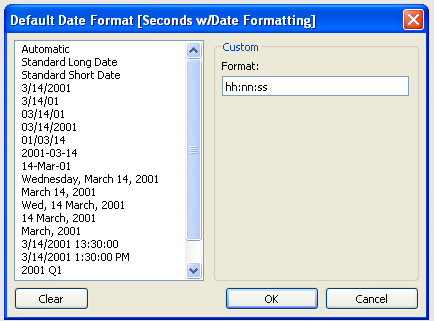Here’s a view…take a look at what happens at 86400 seconds and beyond: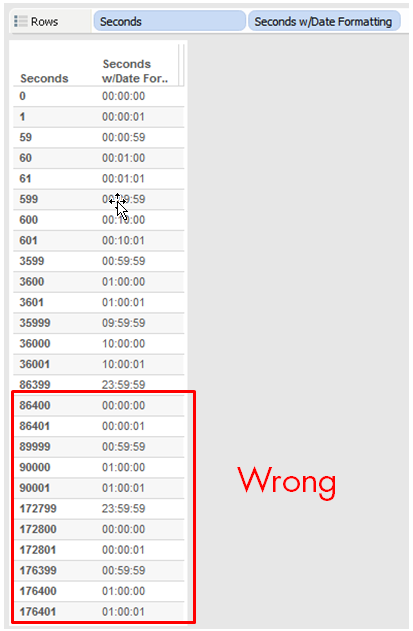Instead of showing 24 hours, the date formatting looks just at the hours, minutes, and seconds. As long as your duration is less than 86400 seconds, everything will be simple using this technique.

# Any Duration: Using Arithmetic and Number Formatting

Though Tableau’s built-in formatting is not as powerful as Excel, we can still do quite a bit. Check out Robert Mundigl’s Custom Number Formats for a pretty exhaustive list of what can be done. (He’s also got a fantastic post on String Calculations that is worth checking out.)

For example, we can set up a custom number format of 00:00, and that will perfectly format a number as a time…up to 59 seconds, that is: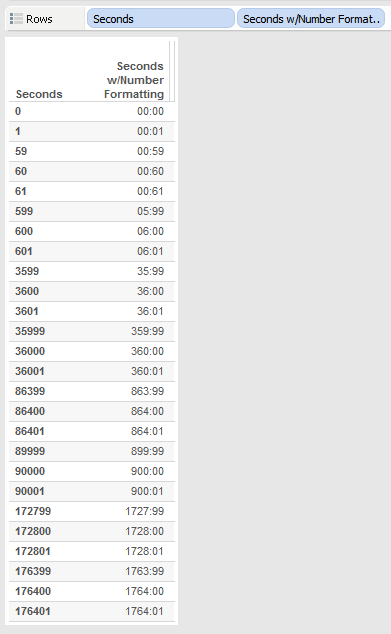However, this is a clue to the next step, which is that since we space out numbers by colons, all we need to do is get the right value into the right decimal place. So, for example, instead of 60 seconds (1 minute) being a value of 60, it has to have a value of 100 so the 00:00 formatting will make it 01:00. Here’s a formula that does just that for mm:ss values:

```//replace [Seconds] with whatever field has the number of seconds in it
//and use a custom number format of 00:00:00:00
//(drop the first 0 to get rid of leading 0's for minutes)
IIF([Seconds] % 60 == 60,0,[Seconds] % 60)// seconds
+ INT([Seconds]/60) * 100 //minutes```
In this formula, [Seconds] is a record-level field. In order for the calculation to work accurately in some situations (such as using it with Subtotals and Grand Totals) you’ll need to change [Seconds] to SUM([Seconds]) or some already-aggregated calculated field. –added 2014-12-22 per notes in the comments.

The formula uses the % (modulo) function to divide the number of seconds by 60 to get the remainder, the IIF statement is there to deal with what happens when the number of seconds is divisible by 60, the result of that is the number of seconds, then the number of minutes *100 is added to that. Here’s a view: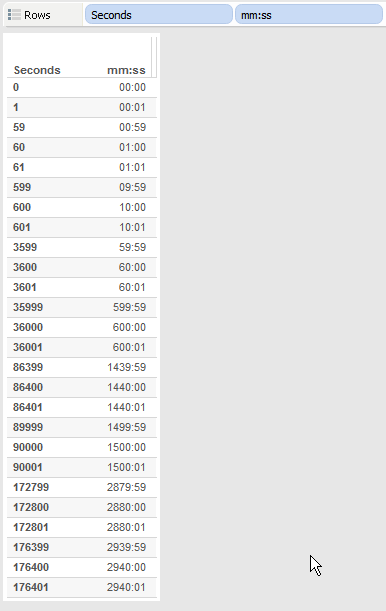For hh:mm:ss the formula gets a little more complex. Just as the seconds had to be transformed to make 0-59 seconds, minutes have to do a similar transformation:

```//replace [Seconds] with whatever field has the number of seconds in it
//and use a custom number format of 00:00:00 (drop the first 0 to get rid of leading 0's for hours)
IIF([Seconds] % 60 == 60,0,[Seconds] % 60)// seconds
+ IIF(INT([Seconds]/60) %60 == 60, 0, INT([Seconds]/60) %60) * 100 //minutes
+ INT([Seconds]/3600) * 10000 //hours```

And for dd:hh:mm:ss there’s yet another transformation to convert hours to 0-23:

```//replace [Seconds] with whatever field has the number of seconds in it
//and use a custom number format of 00:00:00:00 (drop the first 0 to get rid of leading 0's for days)
IIF([Seconds] % 60 == 60,0,[Seconds] % 60)// seconds
+ IIF(INT([Seconds]/60) %60 == 60, 0, INT([Seconds]/60) %60) * 100 //minutes
+ IIF(INT([Seconds]/3600) % 24 == 0, 0, INT([Seconds]/3600) % 24) * 10000 //hours
+ INT([Seconds]/86400) * 1000000 // days```

Here’s a view showing all three calculations: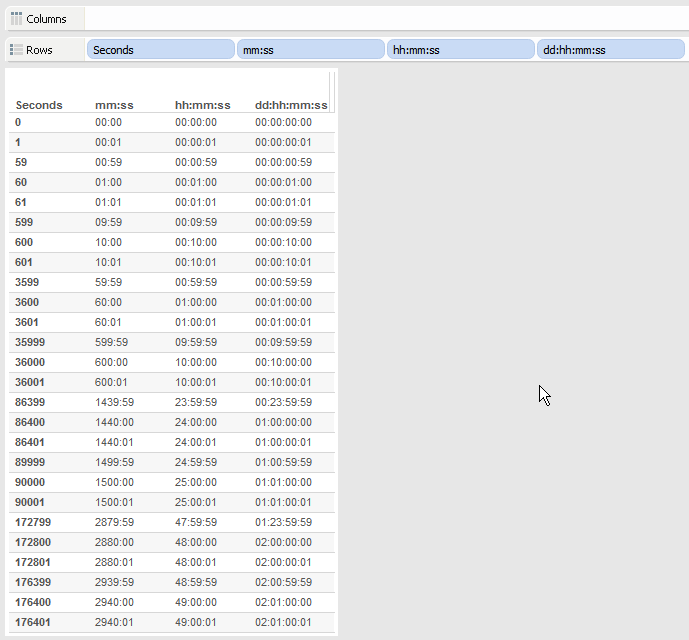A little dose of math, sprinkle some custom number formatting on it, and voila, there’s some usable duration formatting. If you wanted to keep going into weeks then there would need to be another level of calculation, to get into months and years I’d probably use a different approach because the intervals (month lengths and year lengths) aren’t fixed.

Here’s the workbook on Tableau Public: Duration Formatting

If this is useful to you, or you have an alternative technique, let me know in the comments below!

# Addendum on Aggregation (July 2015)

Some people people using this technique have run into problems as seen in the comments below and on the Tableau forums. For example, if we build a view like this and sum up all the values of Seconds with the duration calc applied (see the SUM() aggregation) the numbers don’t add up. In this view the total time in mm:ss should be 44558:00 but it’s showing up as 44554:80, a non-sensical amount: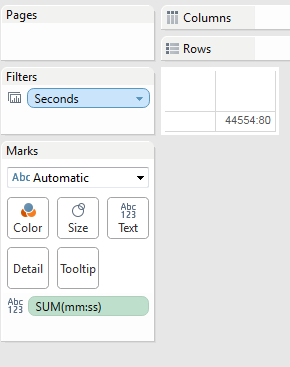Alternatively, if we keep Seconds in the view as a dimension but add a Grand Total, the Grand Total isn’t adding up either:The issue is with regards to order of operations. When we build the views with the grand total or by summing the measure, there’s an aggregation happening and it’s not happening at the right time vis-a-vis the formatting. I put together a series of graphics to explain what is going on.

In the original post the duration calculations are performed for each record and the results are treturned from the view. So it looks like this: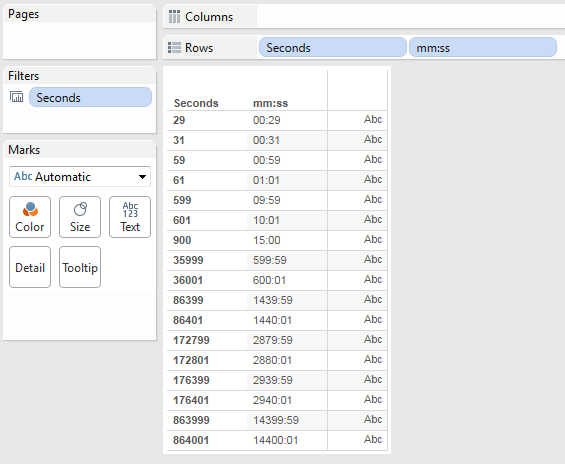So in this case here’s what’s going on under the hood:That’s not often used in practice, more often a view looks like this, where the mm:ss is being used as a measure and then when the original calculation is brought into the view we can see that Tableau is actually aggregating the duration calculation with the default aggregation of SUM():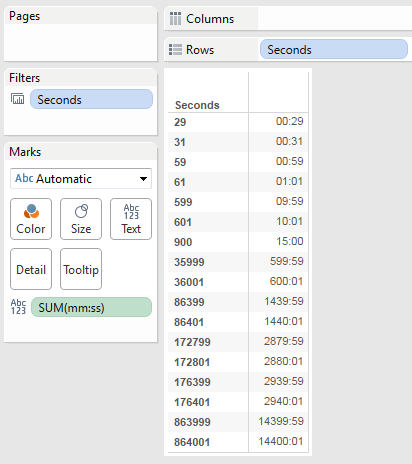However, that SUM() has no effect because the level of detail (the granularity) of the view is the same is the same as the data — in other words, the view is effectively displaying the raw data. So the order of operations is actually like this:So even though these two views look exactly the same, under the hood they are using two different ways to get there. Where this causes problems is when we apply an aggregation that is across multiple records or in a grand total or subtotal. The duration calculation that was meant to be applied to the final result is being applied to every record and it’s that transmogrified amount that is getting summed: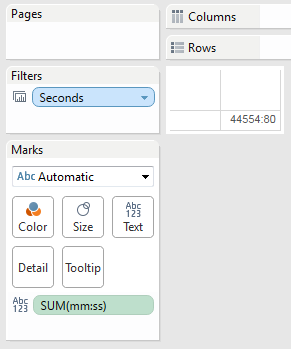The solution is pretty straightforward, we just need to do the aggregation *before* we apply the duration calculation. So instead of the [Seconds] of the original calculation we use Sum Seconds with the formula SUM([Seconds]), and the formula for mm:ss (agg) is:

```//replace [Sum Seconds] with whatever field has the number of seconds in it
IIF([Sum Seconds] % 60 == 60,0,[Sum Seconds] % 60)// seconds
+ INT([Sum Seconds]/60) * 100 //minutes```

So the order of operations is now:And here’s a view showing the inaccurate and now accurate aggregate calculations: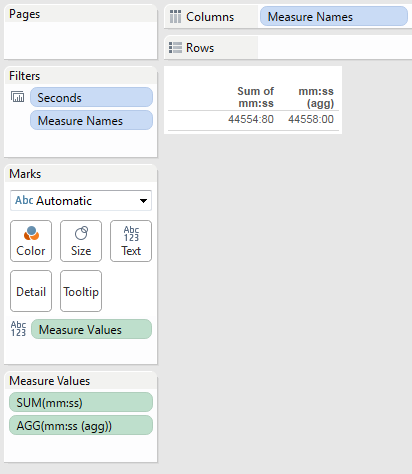And a grand total: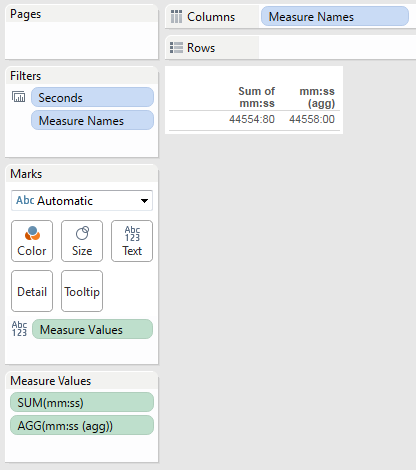I’ve updated the Duration Formatting workbook to reflect this addendum.